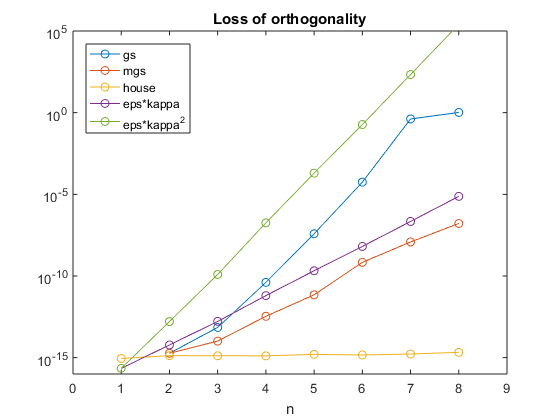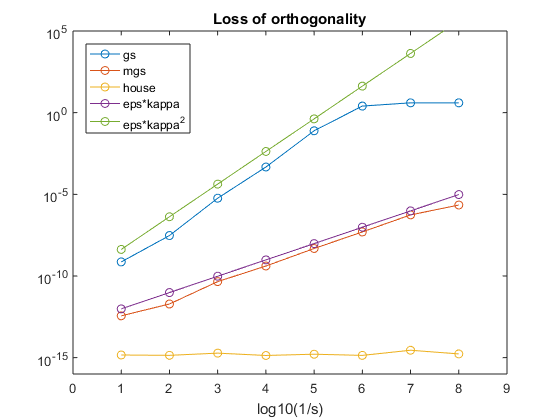# Apologies to Gram-Schmidt

This is a follow-up to my previous follow-up, posted several days ago. A very careful reader, Bruno Bazzano, contributed a comment pointing out what he called "a small typo" in my code for the classic Gram-Schmidt algorithm. It is more than a small typo, it is a serious blunder. I must correct the code, then do more careful experiments and reword my conclusions.

### Contents

#### Classic Gram-Schmidt

Bruno noticed that in the inner loop of my classic Gram-Schmidt function, the expression Q(:,k-1) should be Q(:,1:k-1). I had dropped the two characters, " 1: ". I need to orthogonalize against all the previous vectors, not just one of them. Here is the corrected code.

   type gs

function [Q,R] = gs(X)
% Classical Gram-Schmidt.  [Q,R] = gs(X);
% G. W. Stewart, "Matrix Algorithms, Volume 1", SIAM, 1998.
[n,p] = size(X);
Q = zeros(n,p);
R = zeros(p,p);
for k = 1:p
Q(:,k) = X(:,k);
if k ~= 1
R(1:k-1,k) = Q(:,1:k-1)'*Q(:,k);
Q(:,k) = Q(:,k) - Q(:,1:k-1)*R(1:k-1,k);
end
R(k,k) = norm(Q(:,k));
Q(:,k) = Q(:,k)/R(k,k);
end
end


#### Publishing code

By the way, this points out the importance of publishing codes.

#### Comparison

For various matrices X, let's again check how the three algorithms perform on two tasks, accuracy and orthogonality. How close is Q*R to X? And, how close is Q'*Q to I. I have modified the compare function in last week's post to return ortherr.

#### Well conditioned

First, try a well conditioned matrix, a magic square of odd order.

   n = 7;
X = magic(n)

X =
30    39    48     1    10    19    28
38    47     7     9    18    27    29
46     6     8    17    26    35    37
5    14    16    25    34    36    45
13    15    24    33    42    44     4
21    23    32    41    43     3    12
22    31    40    49     2    11    20


Check its condition.

   kappa = condest(X)

kappa =
8.5681


Do the comparison.

   compare(X);

                 Classic   Modified  Householder
QR error        1.52e-16   6.09e-17   5.68e-16
Orthogonality   1.87e-15   1.53e-15   1.96e-15


The orthogonality value in the Classic column is now at roundoff level. All three algorithms do well with both accuracy and orthogonality on this matrix.

#### Poorly conditioned Hilbert matrices

I want to do two experiments with matrices that are poorly conditioned, but not exactly singular. First, Hilbert matrices of increasing order.

   kappa = zeros(8,1);
ortherr = zeros(8,3);
for n = 1:8
X = hilb(n);
kappa(n) = condest(X);
ortherr(n,:) = compare(X);
end


Plot the results with a logarithmic scale.

    semilogy([ortherr eps*kappa eps*kappa.^2],'o-')
axis([0 9 10^(-16) 10^5])
legend({'gs','mgs','house','eps*kappa','eps*kappa^2'}, ...
'location','northwest')
title('Loss of orthogonality')
xlabel('n')The loss of orthogonality of GS is worse than the other two and roughly varies like the square of the condition number, kappa. The loss of orthogonality of MGS varies like kappa to the first power. Householder does well with orthogonality.

#### Poorly conditioned stabilized magic square

Another set of nonsingular, but poorly conditioned, matrices, generated by adding a small value to the diagonal of the magic square of order 8

   M = magic(8);
I = eye(8);
for k = 1:8
s = 10^(-k);
X = M + s*I;
kappa(k) = condest(X);
ortherr(k,:) = compare(X);
end


Plot the results with a logarithmic scale.

    semilogy([ortherr eps*kappa eps*kappa.^2],'o-')
axis([0 9 10^(-16) 10^5])
legend({'gs','mgs','house','eps*kappa','eps*kappa^2'}, ...
'location','northwest')
title('Loss of orthogonality')
xlabel('log10(1/s)')Again, the orthogonality of GS varies like kappa^2, and the orthogonality of MGS varies like kappa.

#### Singular

Finally, an exactly singular matrix, a magic square of even order.

   n = 8;
X = magic(n);
compare(X);

                 Classic   Modified  Householder
QR error        4.78e-17   8.54e-17   4.85e-16
Orthogonality   5.17e+00   2.16e+00   1.30e-15


All three algorithms do well with accuracy. Both Gram-Schmidts fail completely with orthogonality. Householder still does well with orthogonality.

#### Conclusion

All three of these algorithms provide Q and R that do a good job of reproducing the data X, that is

• Q * R is always close to X for all three algorithms.

On the other hand, their behavior is very different when it comes to producing orthogonality.

• Classic Gram-Schmidt. Loss of orthogonality can be much worse than the other two when X is badly conditioned.
• Modified Gram-Schmidt. Q'*Q varies like the condition of X and fails completely when X is singular.
• Householder triangularization. Q'*Q is always close to I

#### References

G. W. Stewart, Matrix Algorithms: Volume 1: Basic Decompositions, SIAM, xix+458, 1998. <http://epubs.siam.org/doi/book/10.1137/1.9781611971408>

Ake Bjorck, Numerical Methods for Least Squares Problems, SIAM, xvii+407, 1996. <http://epubs.siam.org/doi/book/10.1137/1.9781611971484>

Published with MATLAB® R2016a

|